Miscellaneous

Chapter 1 Class 11 Sets
Serial order wise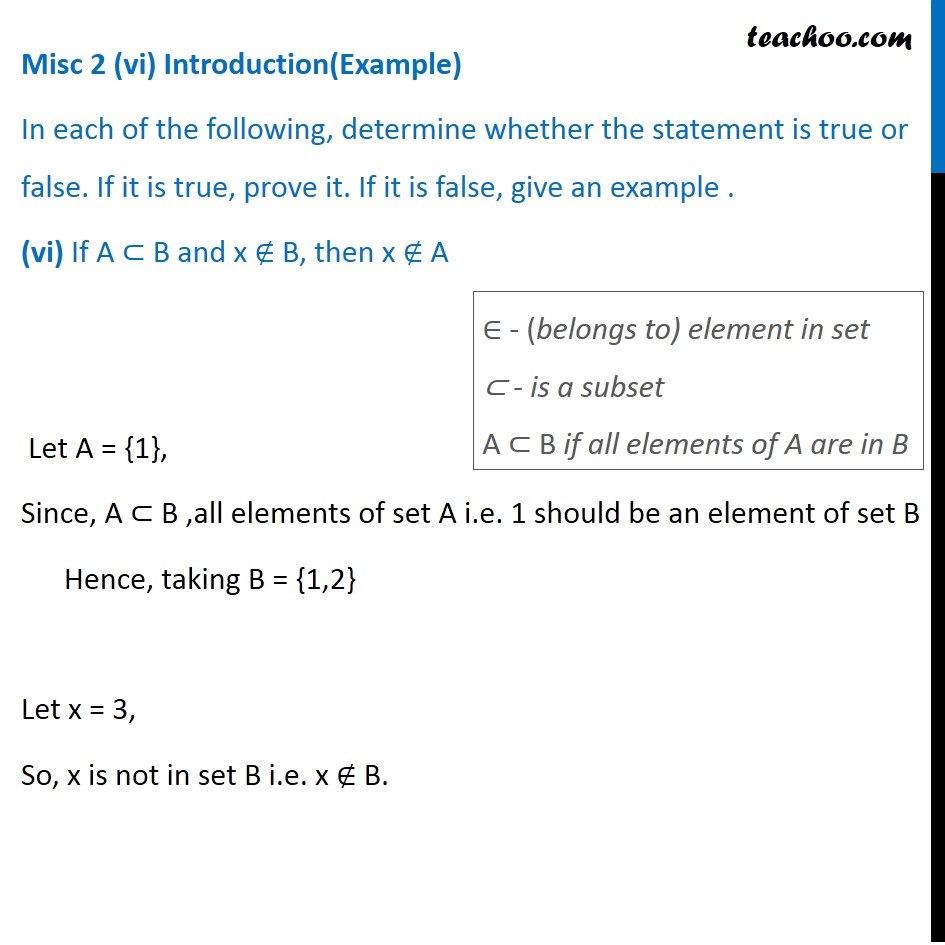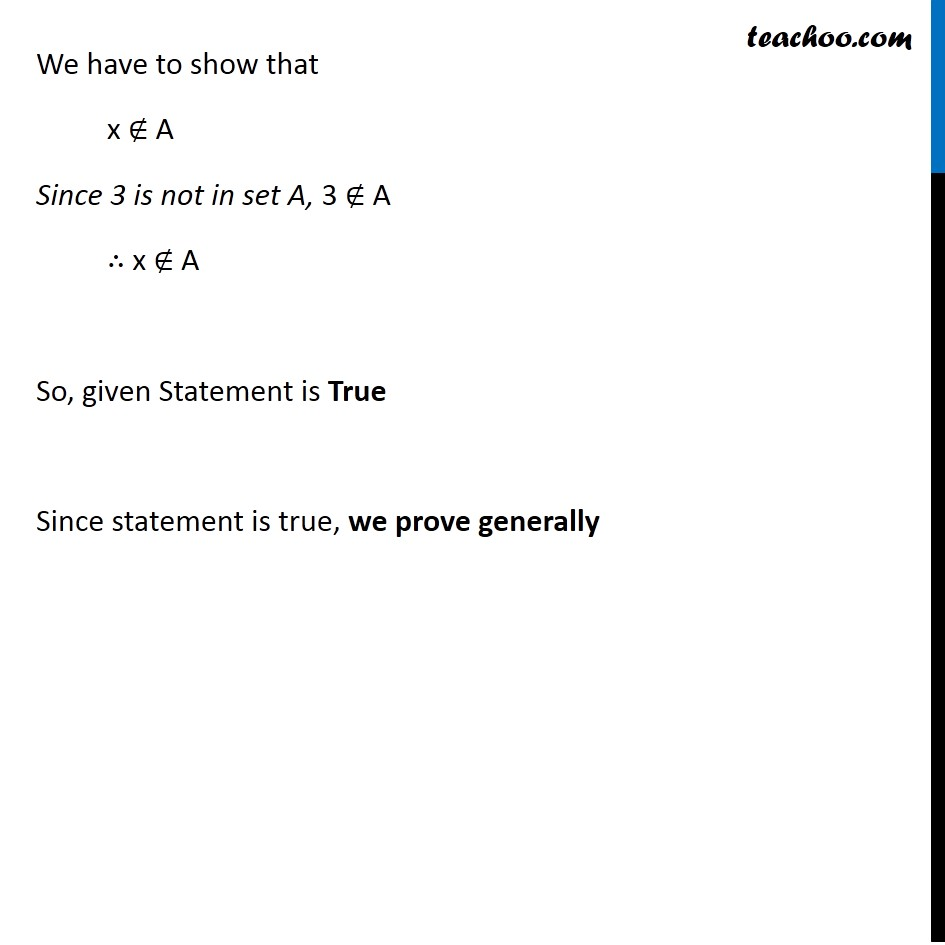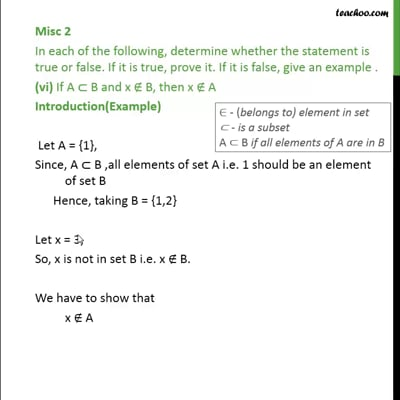This video is only available for Teachoo black users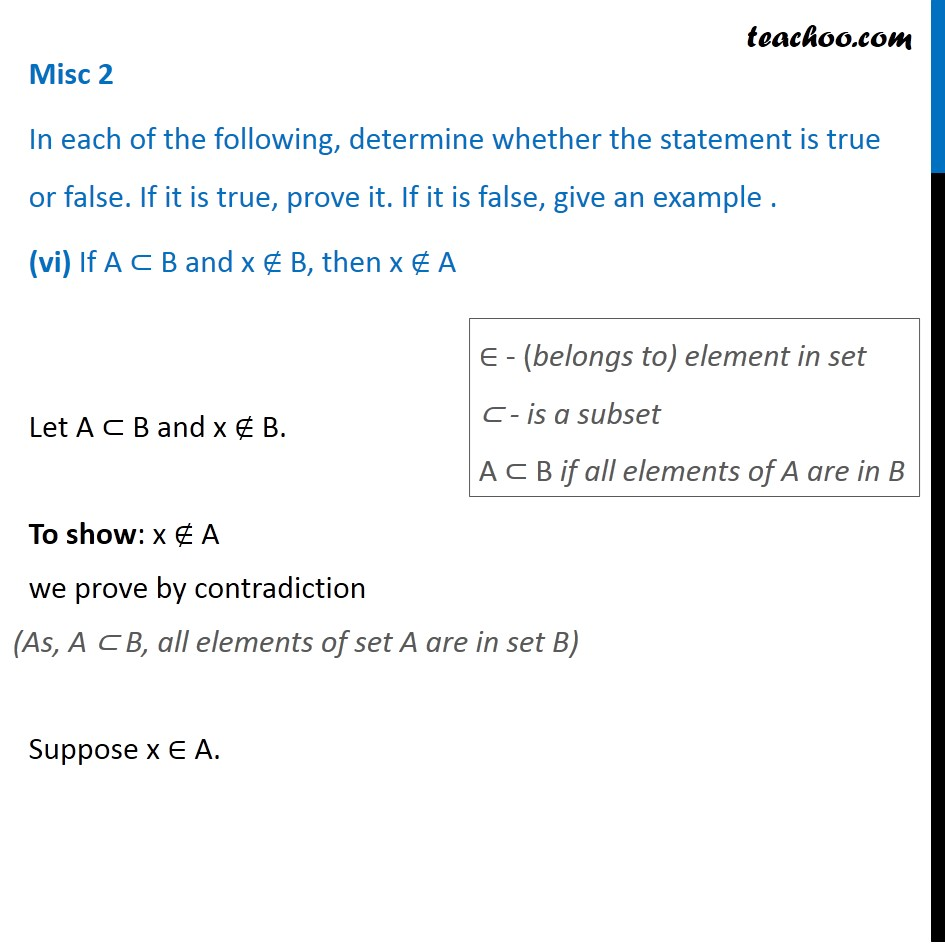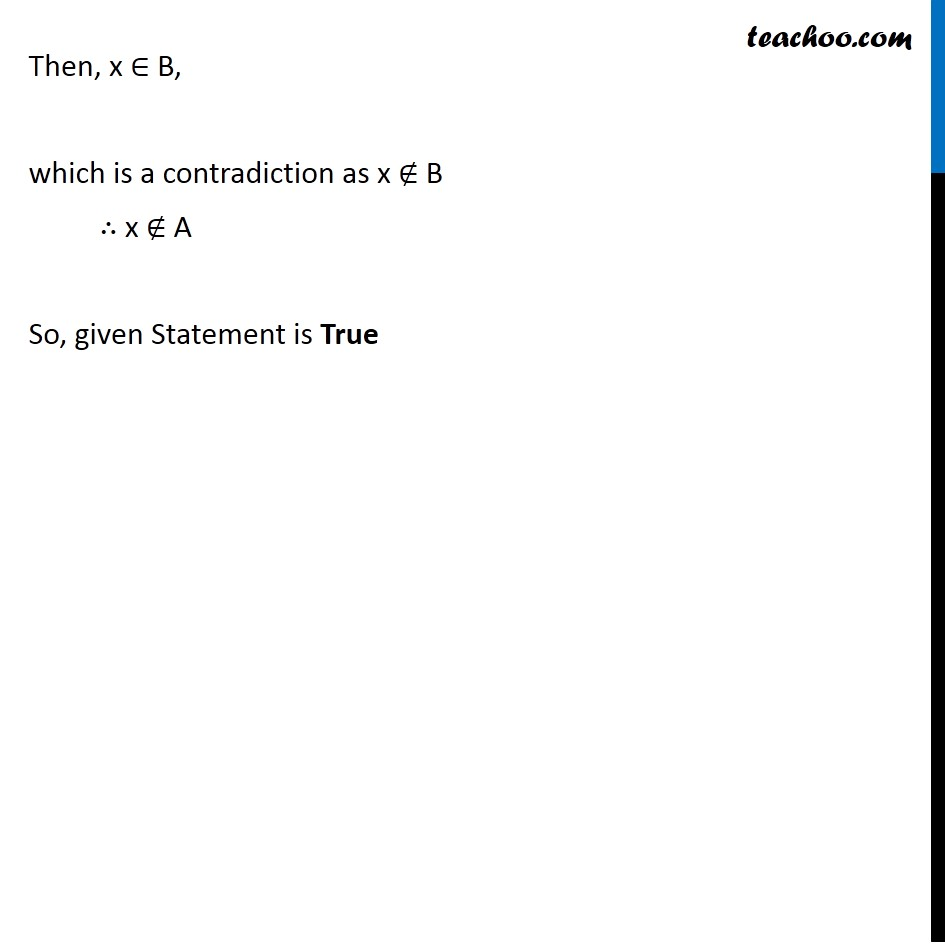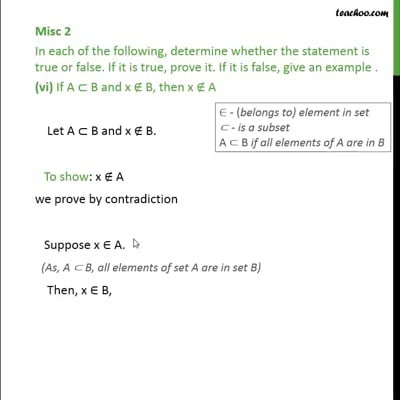This video is only available for Teachoo black users

Introducing your new favourite teacher - Teachoo Black, at only ₹83 per month

### Transcript

Misc 2 (vi) Introduction(Example) In each of the following, determine whether the statement is true or false. If it is true, prove it. If it is false, give an example . (vi) If A ⊂ B and x ∉ B, then x ∉ A Let A = {1}, Since, A ⊂ B ,all elements of set A i.e. 1 should be an element of set B Hence, taking B = {1,2} Let x = 3, So, x is not in set B i.e. x ∉ B. ∈ - (belongs to) element in set ⊂ - is a subset A ⊂ B if all elements of A are in B We have to show that x ∉ A Since 3 is not in set A, 3 ∉ A ∴ x ∉ A So, given Statement is True Since statement is true, we prove generally We have to show that x ∉ A Since 3 is not in set A, 3 ∉ A ∴ x ∉ A So, given Statement is True Since statement is true, we prove generally Misc 2 In each of the following, determine whether the statement is true or false. If it is true, prove it. If it is false, give an example . (vi) If A ⊂ B and x ∉ B, then x ∉ A Let A ⊂ B and x ∉ B. To show: x ∉ A we prove by contradiction Suppose x ∈ A. ∈ - (belongs to) element in set ⊂ - is a subset A ⊂ B if all elements of A are in B Then, x ∈ B, which is a contradiction as x ∉ B ∴ x ∉ A So, given Statement is True